# Problem 3 (20 points) A Bode plot is a graph of the frequency response of a system. It is a combi...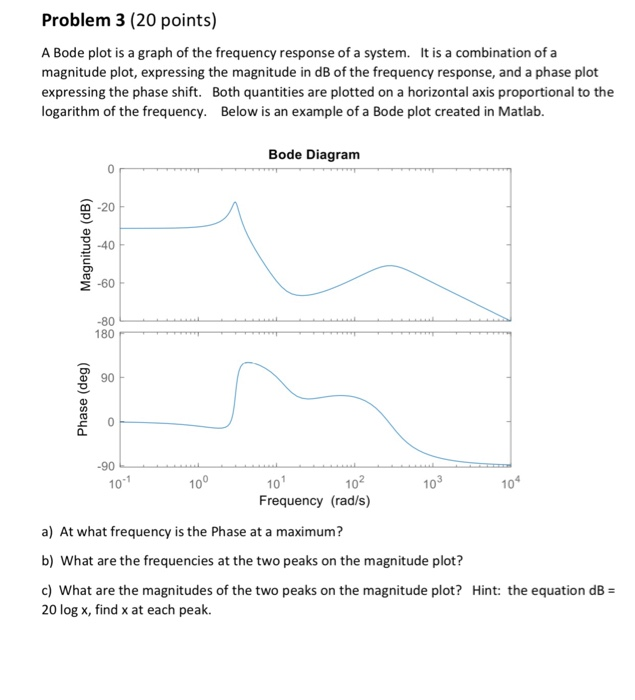Problem 3 (20 points) A Bode plot is a graph of the frequency response of a system. It is a combination of a magnitude plot, expressing the magnitude in dB of the frequency response, and a phase plot expressing the phase shift. Both quantities are plotted on a horizontal axis proportional to the logarithm of the frequency. Below is an example of a Bode plot created in Matlab. Bode Diagram 0 -20 3-40 E-60 -80 180 90 10 10 10 Frequency (rad/s) 10 10 10 a) At what frequency is the Phase at a maximum? b) What are the frequencies at the two peaks on the magnitude plot? c) What are the magnitudes of the two peaks on the magnitude plot? Hint: the equation dB 20 log x, find x at each peak.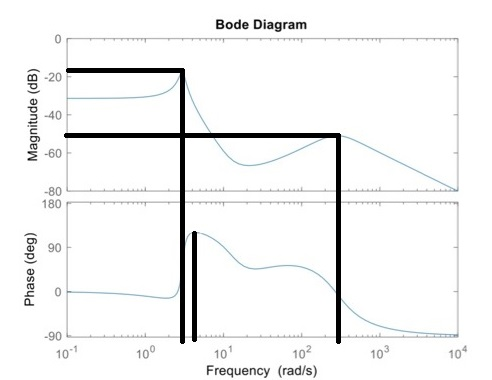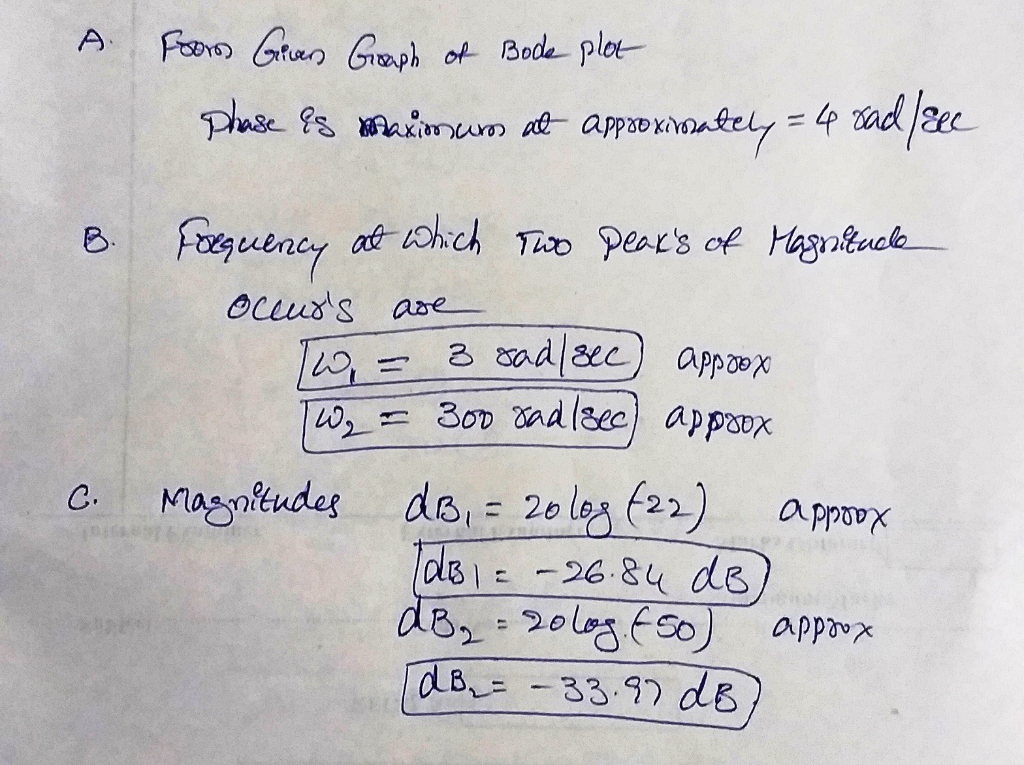##### Add Answer of: Problem 3 (20 points) A Bode plot is a graph of the frequency response of a system. It is a combi...
Similar Homework Help Questions
• ### I need help with this  Bode Plots assignment 2. (5 points) Follow the steps (a-c) described below for the following tra...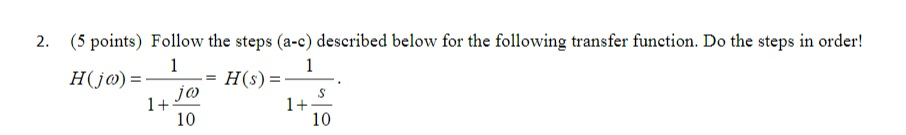I need help with this  Bode Plots assignment 2. (5 points) Follow the steps (a-c) described below for the following transfer function. Do the steps in order! 1+ 10 1+ 10 a. On a 'blank Bode plot' grid, plot the Bode plot straight line hand sketch approximation for magnitude for the frequency range from 1000 to ω.*1000. Be sure, though, that the corner frequency aligns with an appropriate vertical line on the grid. b. On graph paper, directly below the...

• ### I need help with this  Bode Plots assignment (5 points) Follow the steps (a-c) described below for the following transf...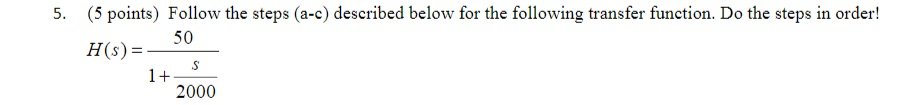I need help with this  Bode Plots assignment (5 points) Follow the steps (a-c) described below for the following transfer function. Do the steps in order! 5. H50 H(s) 2000 a. On a 'blank Bode plot' grid, plot the Bode plot straight line hand sketch approximation for magnitude for the frequency range from 1000 to ω.*1000. Be sure, though, that the corner frequency aligns with an appropriate vertical line on the grid. b. On graph paper, directly below the magnitude...

• ### Q13,Q14 please. 25 For the system with transfer function G(S)  draw the bode (magnitude and s2+4s+25 phase)...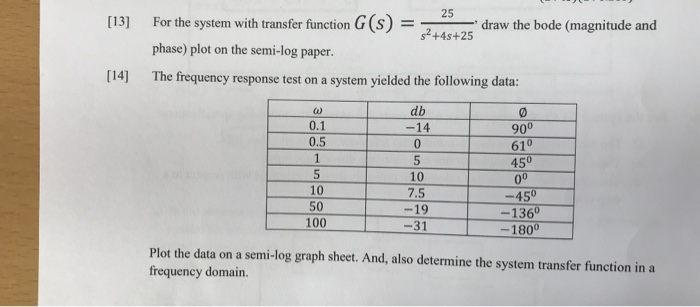Q13,Q14 please. 25 For the system with transfer function G(S)  draw the bode (magnitude and s2+4s+25 phase) plot on the semi-log paper.  The frequency response test ona system yielded the following data: db 0.1 -14 900 610 450 0.5 1 5 5 10 00 10 7.5 -450 50 19 -1360 100 -31 -1800 Plot the data on a semi-log graph sheet. And, also determine the system transfer function in a frequency domain. 25 For the system with transfer...

• ### 3. Find an expression for the step response in the time domain for a system H(s)...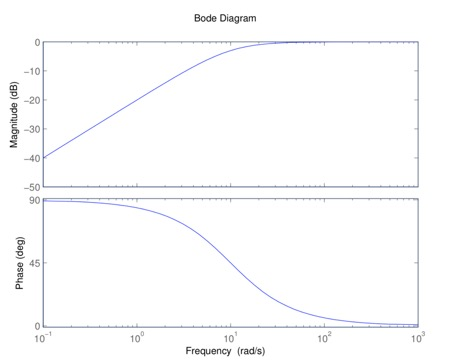3. Find an expression for the step response in the time domain for a system H(s) with Bode diagram shown below. Show all working. Bode Diagram -10 20 -30 -40 -50 90 45 10 10 Frequency (rad/s) 102 10 Magnitude (dB) (Bap) aseud Bode Diagram -10 20 -30 -40 -50 90 45 10 10 Frequency (rad/s) 102 10 Magnitude (dB) (Bap) aseud

• ### Simulation For each filter mentioned in the following cases, first simulate the circuit using Multisim. You can get a plot of the transfer function that is called the Bode plot. From the right toolba...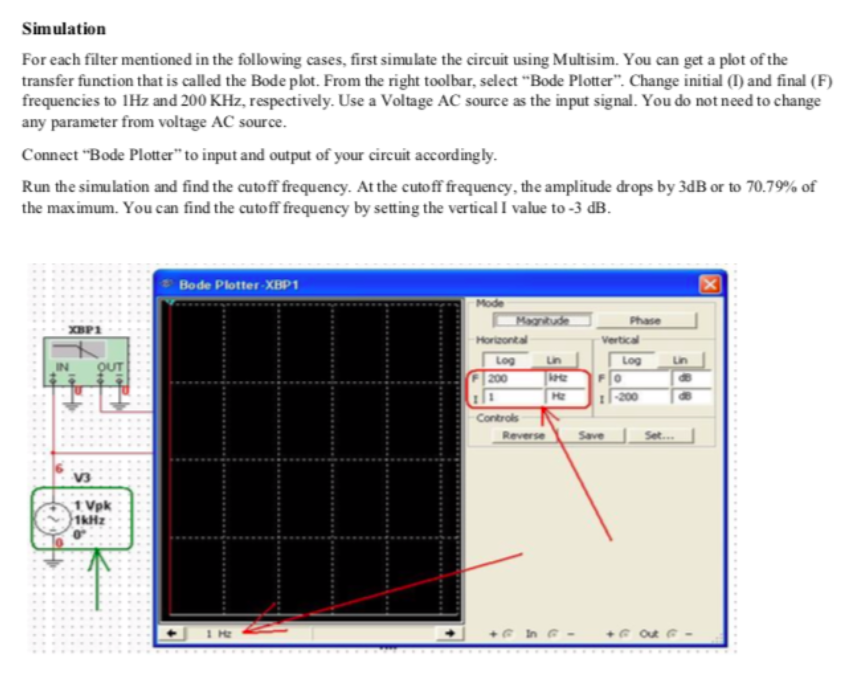Simulation For each filter mentioned in the following cases, first simulate the circuit using Multisim. You can get a plot of the transfer function that is called the Bode plot. From the right toolbar, select "Bode Plotter". Change initial (I) and final (F) frequencies to 1Hz and 200 KHz, respectively. Use a Voltage AC source as the input signal. You do not need to change any parameter from voltage AC source. Connect "Bode Plotter" to input and output of your...

• ### For each filter mentioned in the following cases, first simulate the circuit using Multisim. You can get a plot of the transfer function that is called the Bode plot. From the right toolbar, select &...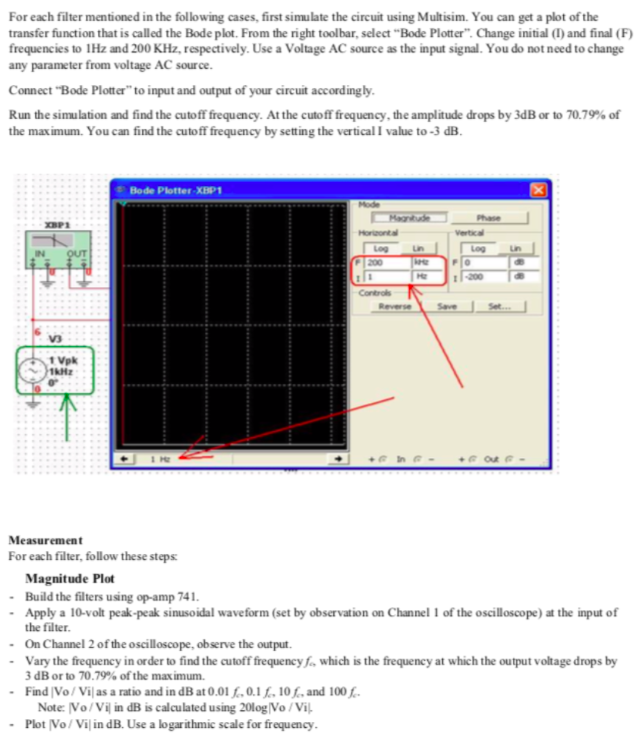For each filter mentioned in the following cases, first simulate the circuit using Multisim. You can get a plot of the transfer function that is called the Bode plot. From the right toolbar, select "Bode Plotter". Change initial (I) and final (F frequencies to 1Hz and 200 KHz, respectively. Use a Voltage AC source as the input signal. You do not need to change any parameter from voltage AC source Connect "Bode Plotter" to input and output of your circuit...

• ### 3. You are given a system transfer function belowl 20 points): 100 (1 +0.001 je) TF(a) a. Convert...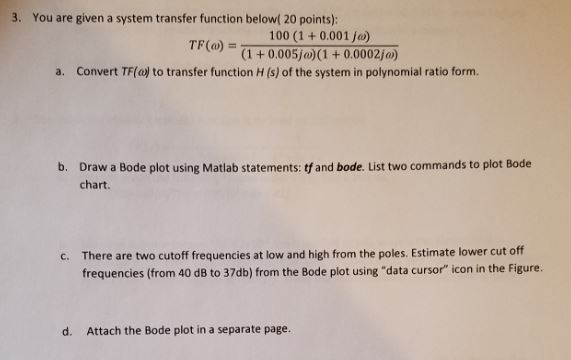3. You are given a system transfer function belowl 20 points): 100 (1 +0.001 je) TF(a) a. Convert TF(o) to transfer function H (s) of the system in polynomial ratio form. Draw a Bode plot using Matlab statements: tf and bode. List two commands to plot Bode chart. b. There are two cutoff frequencies at low and high from the poles. Estimate lower cut off frequencies (from 40 dB to 37db) from the Bode plot using "data cursor" icon in...

• ### please show steps 5. GH(s) is a minimum-phase system which has the Bode plot shown below. It is desired to increase the phase margin by 40 degrees and also increase the closed-loop system bandwidth....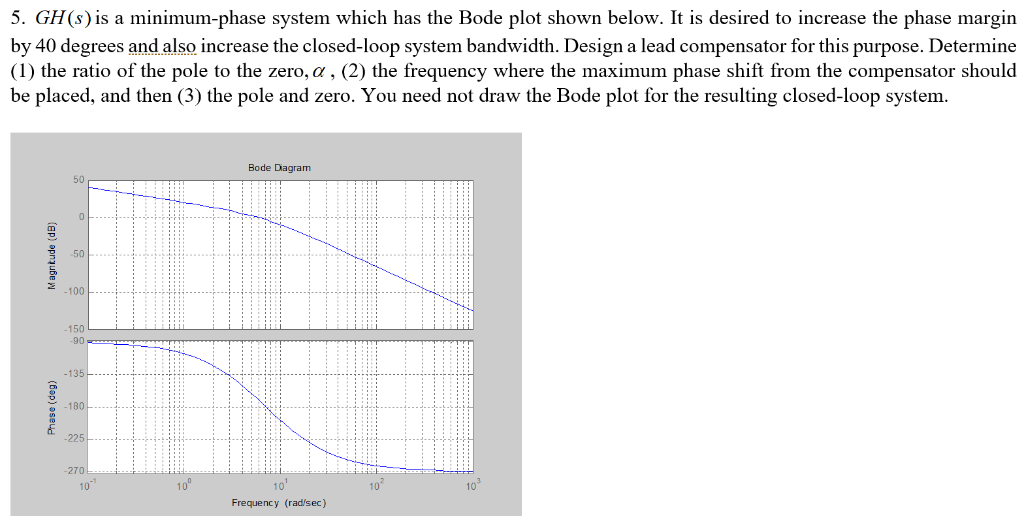please show steps 5. GH(s) is a minimum-phase system which has the Bode plot shown below. It is desired to increase the phase margin by 40 degrees and also increase the closed-loop system bandwidth. Design a lead compensator for this purpose. Determine (1) the ratio of the pole to the zero, α , (2) the frequency where the maximum phase shift from the compensator should be placed, and then (3) the pole and zero. You need not draw the Bode...

• ### For the system transfer function given by: s +10 \$2 x (82100.+10) 1. Identify each term in the tr...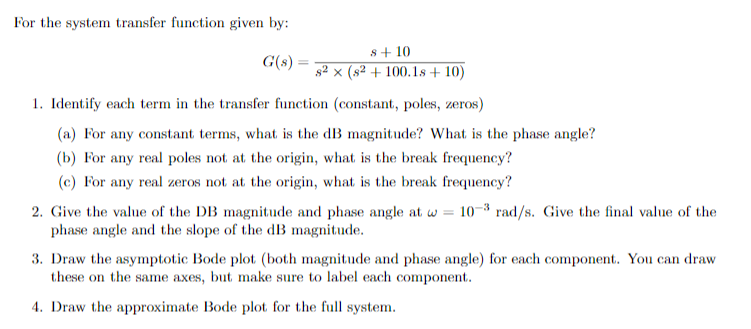For the system transfer function given by: s +10 \$2 x (82100.+10) 1. Identify each term in the transfer function (constant, poles, zeros) (a) For any constant terms, what is the dB magnitude? What is the phase angle? (b) For any real poles not at the origin, what is the break frequency? (c) For any real zeros not at the origin, what is the break frequency? 2. Give the value of the DB magnitude and phase angle at w =...

• ### 40 20 20 -60 -80 -100 0.1 100 10 Frequency (radis) -50 -100 -150 200 250...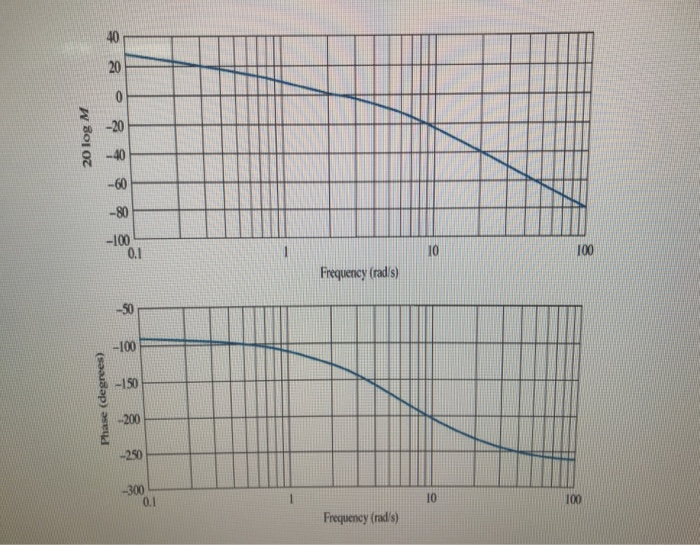40 20 20 -60 -80 -100 0.1 100 10 Frequency (radis) -50 -100 -150 200 250 -300 0.1 10 100 Frequency (rad/s) Phase (degrees) 20 log M O Pictuve UShg upon Bode Plot of KGIS) Closed lop syStem's chaactentic eguation is Des)= 1+KG(S) (4) uhg Magnitude Plot, Detamine the torm of Transfer fevction from slope of HigL fretueney, Slope t low freguency, Cut-aft fefuency an Sretch the Voot -locuS t(wro Miw) 20d8 Vampng atto =1) on a seand -order System)...

Need Online Homework Help?